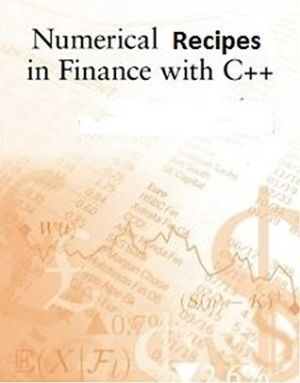# Financial Numerical Recipes in C++

by Bernt Arne ØdegaardDescriptionTable of ContentsDetailsHashtagsReport an issue

### Book Description

This book is a a discussion of the calculation of specific formulas in finance. The field of finance has seen a rapid development in recent years, with increasing mathematical sophistication. While the formalization of the field can be traced back to the work of Markowitz (1952) on investors mean-variance decisions and Modigliani and Miller (1958) on the capital structure problem, it was the solution for the price of a call option by Black and Scholes (1973); Merton (1973) which really was the starting point for the mathematicalization of finance. The fields of derivatives and fixed income have since then been the main fields where complicated formulas are used. This book is intended to be of use for people who want to both understand and use these formulas, which explains why most of the algorithms presented later are derivatives prices.

This project started when I was teaching a course in derivatives at the University of British Columbia, in the course of which I sat down and wrote code for calculating the formulas I was teaching. I have always found that implementation helps understanding these things. For teaching such complicated material it is often useful to actually look at the implementation of how the calculation is done in practice. The purpose of the book is therefore primarily pedagogical, although I believe all the routines presented are correct and reasonably efficient, and I know they are also used by people to price real options.

To implement the algorithms in a computer language I choose C++. My students keep asking why anybody would want to use such a backwoods computer language, they think a spreadsheet can solve all the worlds problems. I have some experience with alternative systems for computing, and no matter what, in the end you end up being frustrated with higher end "languages", such as Matlab og R (Not to mention the straitjacket which is is a spreadsheet.) and going back to implementation in a standard language. In my experience with empirical finance I have come to realize that nothing beats knowledge a real computer language. This used to be FORTRAN, then C, and now it is C++. All example algorithms are therefore coded in C++. I do acknowledge that matrix tools like Matlab are very good for rapid prototyping and compact calculations, and will in addition to C++ in places also illustrate the use of Matlab, as well as other (public domain) tools.

This open book is licensed under a Open Publication License (OPL). You can download Financial Numerical Recipes in C++ ebook for free in PDF format (1.4 MB).

### Table of Contents

Chapter 1
On C++ and programming
Chapter 2
Matrix Tools
Chapter 3
The value of time
Chapter 4
Bond Pricing with a flat term structure
Chapter 5
The term structure of interest rates and an object lesson
Chapter 6
The Mean Variance Frontier
Chapter 7
Futures algoritms
Chapter 8
Binomial option pricing
Chapter 9
Basic Option Pricing, the Black Scholes formula
Chapter 10
Warrants
Chapter 11
Extending the Black Scholes formula
Chapter 12
Option pricing with binomial approximations
Chapter 13
Finite Differences
Chapter 14
Option pricing by simulation
Chapter 15
Pricing American Options - Approximations
Chapter 16
Average, lookback and other exotic options
Chapter 17
Generic binomial pricing
Chapter 18
Trinomial trees
Chapter 19
Alternatives to the Black Scholes type option formula
Chapter 20
Pricing of bond options, basic models
Chapter 21
Credit risk
Chapter 22
Term Structure Models
Chapter 23
Binomial Term Structure models
Chapter 24
Interest rate trees
Chapter 25
Building term structure trees using the Ho and Lee (1986) approach
Chapter 26
Term Structure Derivatives
Chapter 27
Date (and time) revisited - the BOOST libraries

### Book Details

Subject
Computer Science
Publisher
Self-publishing
Published
2014
Pages
264
Edition
1
Language
English
PDF Size
1.4 MB
License
Open Publication License

### Related BooksThere are plenty of books that teach introductory data structures. Some of them are very good. Most of them cost money, and the vast majority of computer science undergraduate students will shell out at least some cash on a data structures book. Open Data Structures (in C++) - The goal of this project is to free undergraduate computer science stud...This book presents computer programming as a key method for solving mathematical problems. This second edition of the well-received book has been extensively revised: All code is now written in Python version 3.6 (no longer version 2.7). In addition, the two first chapters of the previous edition have been extended and split up into five new chapte...This book presents computer programming as a key method for solving mathematical problems. There are two versions of the book, one for MATLAB and one for Python. The book was inspired by the Springer book TCSE 6: A Primer on Scientific Programming with Python (by Langtangen), but the style is more accessible and concise, in keeping with the needs o...This book presents computer programming as a key method for solving mathematical problems. There are two versions of the book, one for MATLAB and one for Python. The book was inspired by the Springer book TCSE 6: A Primer on Scientific Programming with Python (by Langtangen), but the style is more accessible and concise, in keeping with the needs o...Intermediate Financial Accounting Volume 1 developed in collaboration by Athabasca University and Lyryx, is intended for a first course in Intermediate Financial Accounting, and presumes that students have already completed one or two Introductory Financial Accounting courses. The textbook reflects current International Financial Reporting Standard...Introduction to Financial Accounting is intended for a first course in introductory financial accounting. It has been extensively edited by Athabasca University and reflects current International Financial Reporting Standards (IFRS). A corporate approach is utilized versus beginning with a sole proprietorship emphasis and then converting to a corpo...